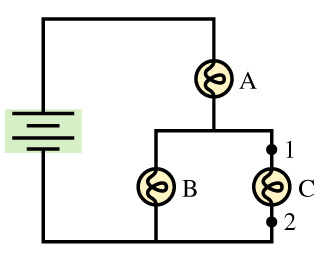# Problem: The three bulbs in the figure are identical.(a) Rank the bulbs from brightest to dimmest.Now, suppose a wire is connected between points 1 and 2.(b) What happens to bulb A?(c) What happens to bulb B?(d) What happens to bulb C?

###### FREE Expert Solution

(a) The bulbs have equal resistance, R. The current through bulb A is divided amongst bulbs B and C equally since they have equal resistance.

Power,

$\overline{){\mathbf{P}}{\mathbf{=}}{{\mathbf{I}}}^{{\mathbf{2}}}{\mathbf{R}}}$

Brightness is directly proportional to the power dissipated by a bulb. The current through each bulb will determine the power dissipated by the bulb relative to the others.

93% (473 ratings)###### Problem Details

The three bulbs in the figure are identical.
(a) Rank the bulbs from brightest to dimmest.
Now, suppose a wire is connected between points 1 and 2.
(b) What happens to bulb A?
(c) What happens to bulb B?
(d) What happens to bulb C?